﻿ Supersonic flow around a triangular prism - XSim

# Supersonic flow around a triangular prism

Update: November 26, 2018
OpenFOAM 4.x

## Case directory

\$FOAM_TUTORIALS/compressible/sonicFoam/ras/prism

## Summary

We calculate the supersonic air flow around a triangular prism.

The Fluid flows in from the region "inlet" at 650 m/s and out from the region "outlet". The length of the flow path from the region "inlet" to the region "outlet" is 1 m. The flow is assumed to be free-flowing in the regions "topWall" and "bottomWall", which are parallel to the flow, and no-slip wall in the region "prismWall", which is a triangular prism surface.Model geometry

The calculation is performed as a 2-dimensional analysis for 0.0004 seconds. The standard k-ε model is used as turbulence model.

The meshes are as follows, and the number of mesh is 6636.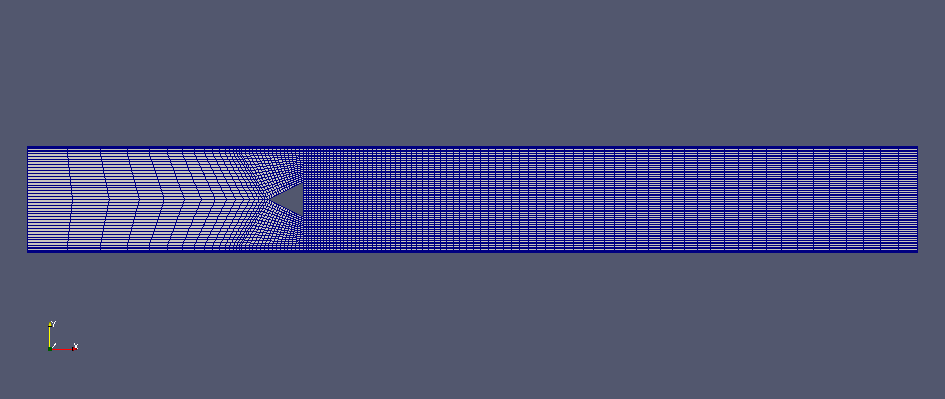Entire meshMesh around the triangular prism

The calculation result is as follows.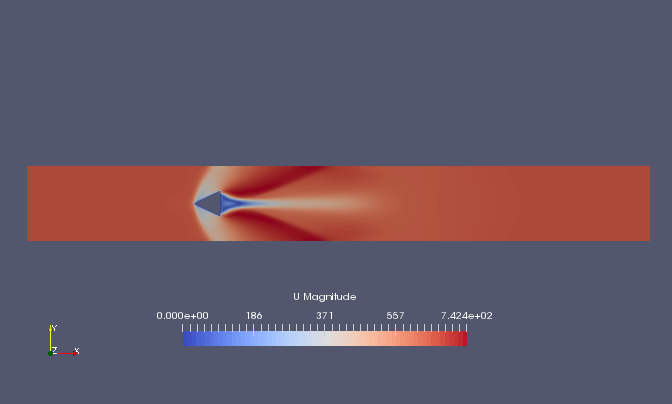Flow velocity at final time (U)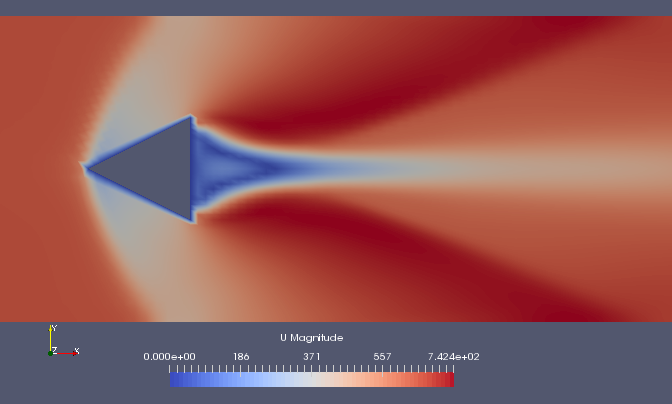Flow Velocity around the triangular prism at the final time (U)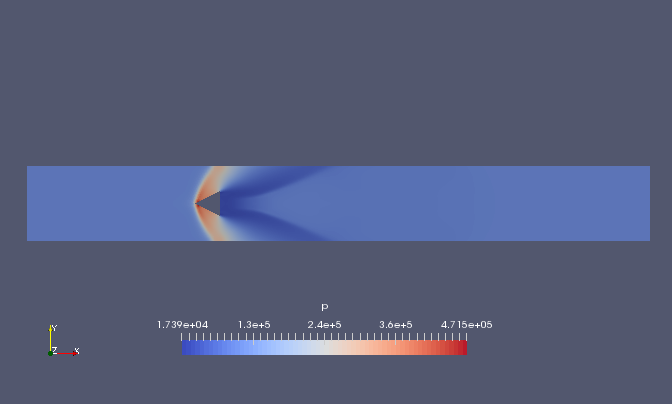Pressure at final time (p)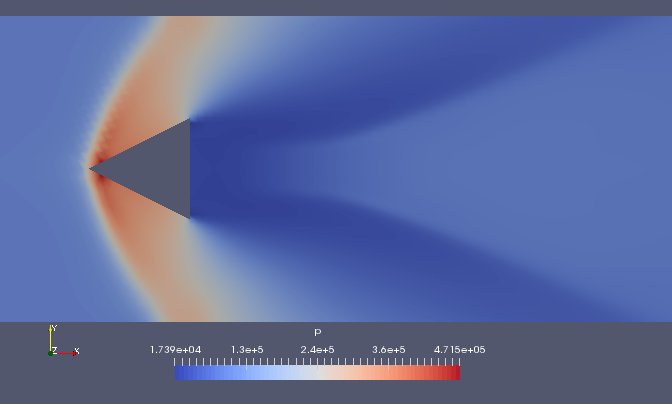Pressure around the triangular prism at the final time (p)

## Commands

cp -r \$FOAM_TUTORIALS/compressible/sonicFoam/ras/prism prism
cd prism

blockMesh
sonicFoam

paraFoam

## Calculation time

12.71 seconds *Single, Inter(R) Core(TM) i7-8700 CPU @ 3.20GHz 3.19GHz